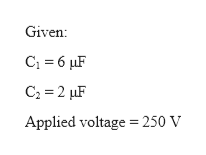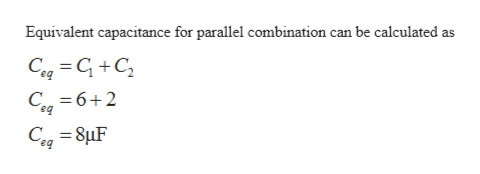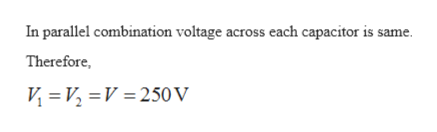# Capacitors C1 = 6.0 µF and C2 = 2.0 µF are charged as a parallelcombination across a 250 - V battery. The capacitors aredisconnected from the battery and from each other. They arethen connected positive plate to negative plate and negativeplate to positive plate. Calculate the resulting charge on eachcapacitor.

Question
12 views

Capacitors C1 = 6.0 µF and C2 = 2.0 µF are charged as a parallel
combination across a 250 - V battery. The capacitors are
disconnected from the battery and from each other. They are
then connected positive plate to negative plate and negative
plate to positive plate. Calculate the resulting charge on each
capacitor.

check_circle

Step 1

Given:

C1 = 6 μF

C2 = 2 μF

Applied voltage = 250 V

Given:

C1 = 6 μF

C2 = 2 μF

Applied voltage = 250 V

Given:

C1 = 6 μF

C2 = 2 μF

Applied voltage = 250 V

Given:

C1 = 6 μF

C2 = 2 μF

Applied voltage = 250 Vhelp_outlineImage TranscriptioncloseGiven: C; = 6 µF C2 = 2 µF Applied voltage = 250 V fullscreen
Step 2help_outlineImage TranscriptioncloseEquivalent capacitance for parallel combination can be calculated as Cag = G +C, C, =6+2 C = 8µF %3D fullscreen
Step 3help_outlineImage TranscriptioncloseIn parallel combination voltage across each capacitor is same. Therefore, V = V, =V = 250 V fullscreen

### Want to see the full answer?

See Solution

#### Want to see this answer and more?

Solutions are written by subject experts who are available 24/7. Questions are typically answered within 1 hour.*

See Solution
*Response times may vary by subject and question.
Tagged in
SciencePhysics

### Electrostatic Potential and Capacitance